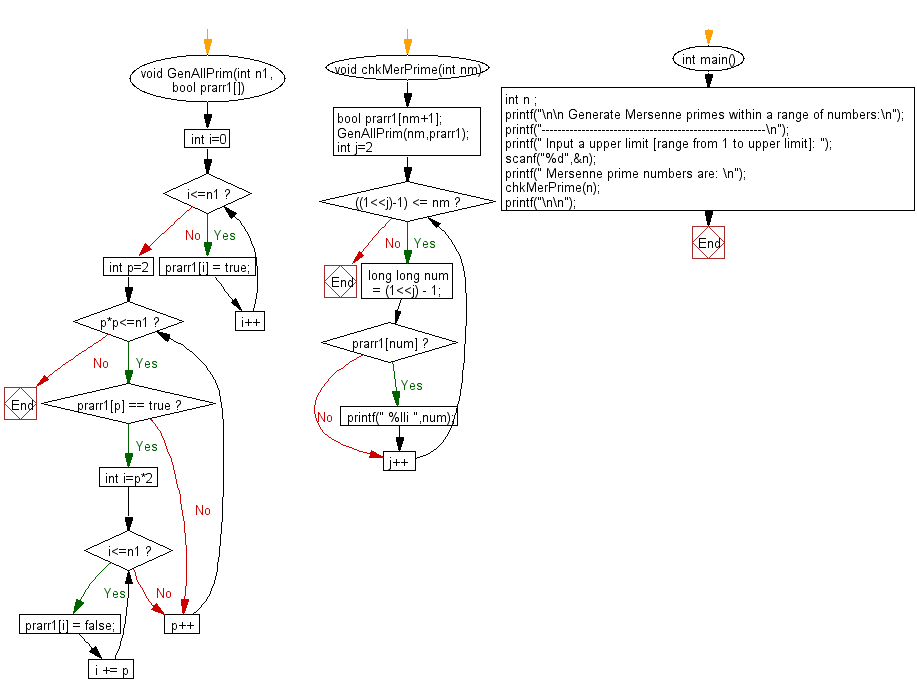﻿ C Program: Mersenne primes within a range of numbers# C Exercises: Generate mersenne primes within a range of numbers

## C Numbers: Exercise-33 with Solution

Write a program in C to generate mersenne primes within a range of numbers.

Test Data
Input a upper limit [range from 1 to upper limit]: 1000

Sample Solution:

C Code:

``````# include <stdio.h>
# include <stdlib.h>
# include <stdbool.h>
# include <math.h>

void GenAllPrim(int n1, bool prarr1[])
{
for (int i=0; i<=n1; i++)
prarr1[i] = true;

for (int p=2; p*p<=n1; p++)
{
if (prarr1[p] == true)
{
for (int i=p*2; i<=n1; i += p)
prarr1[i] = false;
}
}
}
void chkMerPrime(int nm)
{
bool prarr1[nm+1];
GenAllPrim(nm,prarr1);
for (int j=2; ((1<<j)-1) <= nm; j++)
{
long long num = (1<<j) - 1;
if (prarr1[num])
printf(" %lli ",num);
}
}
int main()
{
int n ;
printf("\n\n Generate Mersenne primes within a range of numbers:\n");
printf("--------------------------------------------------------\n");
printf(" Input a upper limit [range from 1 to upper limit]: ");
scanf("%d",&n);
printf(" Mersenne prime numbers are: \n");
chkMerPrime(n);
printf("\n\n");
}
```
```

Sample Output:

``` Input a upper limit [range from 1 to upper limit]: 1000
Mersenne prime numbers are:
3  7  31  127
```

Pictorial Presentation:Flowchart:C Programming Code Editor:

What is the difficulty level of this exercise?

Test your Programming skills with w3resource's quiz.

﻿

## C Programming: Tips of the Day

Why do C and C++ compilers allow array lengths in function signatures when they're never enforced?

It is a quirk of the syntax for passing arrays to functions.

Actually it is not possible to pass an array in C. If you write syntax that looks like it should pass the array, what actually happens is that a pointer to the first element of the array is passed instead.

Since the pointer does not include any length information, the contents of your [] in the function formal parameter list are actually ignored.

Ref : https://bit.ly/3fhlvdH